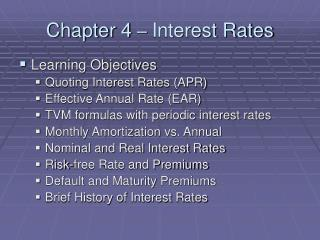DownloadDownload PresentationChapter 4 – Interest Rates

# Chapter 4 – Interest Rates

Download Presentation## Chapter 4 – Interest Rates

- - - - - - - - - - - - - - - - - - - - - - - - - - - E N D - - - - - - - - - - - - - - - - - - - - - - - - - - -
##### Presentation Transcript

1. Chapter 4 – Interest Rates • Learning Objectives • Quoting Interest Rates (APR) • Effective Annual Rate (EAR) • TVM formulas with periodic interest rates • Monthly Amortization vs. Annual • Nominal and Real Interest Rates • Risk-free Rate and Premiums • Default and Maturity Premiums • Brief History of Interest Rates

2. Annual and Periodic Rates • Annual Percentage Rate (APR) • The standard way to quote interest rate • Actual rate may be different • Period or Periodic Interest Rate • The rate for the period • Quarterly, Monthly, Daily, etc. • Annual Percentage Rate divided by Compounding periods per year • Effective Annual Rate (EAR) is the periodic rate compounded over a year

3. Annual and Periodic Rates • Periodic Rates with 5.0% APR • Semi-Annual Rate (compounds twice a year) • 5.0% / 2 = 2.5% • Monthly Rate (compounds twelve times a year) • 5.0% / 12 = 0.04167% • Effective Annual Rates • EAR = (1 + [APR / (C/Y)])C/Y – 1, equation 4.2 • Semi-Annual Rate of 2.5%, EAR = 5.0625% • Monthly Rate of 0.04167%, EAR = 5.1162%

4. Annual and Periodic Rates • Effective Annual Rate is the rate that you earn with your investment and it increases with the number of compounding periods per year (C/Y) • Maximum compounding is continuous compounding and • EAR = eAPR -1 • EAR = e0.05 -1= 5.12711% • e is the exponential function

5. Impact on TVM • The r in the TVM equations is the periodic rate • It is the APR when compounding is annually • The n in the TVM equation matches r and is the number of periods (compounding periods per year times number of years) • Changing calculator mode for P/Y and C/Y

6. Impact on TVM • Increasing the number of compounding periods changes results (changes effective interest rate) • Basic Mortgage Payment (Interest and Principal as you go on ordinary annuity) • Example 4.1 page 80 • Loan Amount \$190,000 (PV) • Interest quoted at 8% APR • Payments are monthly (ordinary annuity) for 30 years or 360 monthly payments • Monthly Payment for P & I is \$1,394.15

7. Impact on TVM • Example 4.2 – Annual compounding versus Monthly compounding • \$1,000,000 retirement goal at 9% APR • 30 years to retirement • Annual Payment with annual compounding, \$7,336.35 • Monthly Payment with monthly compounding, \$546.23 (\$546.23 x 12 = \$6,554.76) • EAR difference, Annual is 9%, Monthly is 9.381%

8. Consumer Loans and Monthly Amortization Schedule • Most Consumer Loans are annuity stream fixed payments with interest accruing at end of month • Payment is for monthly interest expense and remainder is for principal reduction • Use TVM equation with monthly interest rate as r, number of payments or periods as n, and the amount of the loan as PV to find the monthly payment required.

9. Consumer Loans and Monthly Amortization Schedule • Monthly amortization schedule for car loan of \$25,000 for 72 months at 8% APR (Table 4.3)

10. Nominal and Real Interest Rates • APR and Periodic Rates are nominal rates • Nominal Rates have two components • Real Rate • Expected Inflation Rate • Nominal ≈ Real Rate + Expected Inflation • Real Rate is reward for saving • Example 4.5 – 21 books next year versus 20 books now • Real Rate = 21/20 – 1 = 5% • Expected Inflation is the rising price of a good

11. Nominal and Real Interest Rates • Fisher Effect • Relationship between real rate, expected inflation, and nominal rate • (1+r) = (1+r*) x (1+h) • r is the nominal rate • r* is the real rate • h is expected inflation • r = r* + h + (r* x h) • r* is the same the world round

12. Risk-free Rate and Premiums • Risk-free rate (a nominal or real rate) with a guaranteed return • Nominal risk-free rate such as Treasury Bill • Real risk-free rate (excludes inflation) • Premiums impact the interest rates on different types of investments • Default Risk • Maturity

13. Risk-free Rate and Premiums • Default Risk • Different Investments have different default risk based on the issuers ability to meet future promised payments • Low risk – U.S. Government • High risk – New Start-up Company • Maturity Premium – Investors demand more compensation for waiting longer

14. Risk-free Rate and Premiums • Summary of Interest Rates • TVM equation uses a period nominal interest rate • The nominal interest rate is made up of two components, the real rate and inflation • Different investments have different nominal rates due to potential default (dp), and maturity (mp) • r ≈ rf* + inflation + dp + mp

15. Brief History of Interest Rates • Four Different Investments over 50 Years • 3-Month Treasury Bill (risk-free rate) • Range 1% to 15% • Inflation in United States • Range -0.5% to 13% • Long-Term Treasury Bonds • Range -9% to 32% • Large U.S. Company Stocks • Range -27% to 52%

16. Homework • Problem 3 – Effective Annual Rate • Problem 6 – PV with Periodic Rates • Problem 12– Amortization Schedule • Problem 18 – Nominal Rate